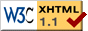Line Breaking

# Line Breaking

2021-04-30

## Introduction

When laying out text on a page, decisions need to be made about how to position the text in a paragraph. In general, it will not be possible or desirable to fit the entire paragraph on a single line, so the paragraph will have to be broken into multiple lines. There are many factors that influence how aesthetically pleasing the result is. This essay explores some of them.

## Scope

Breaking paragraphs into lines is a problem that can be solved very simply, but vastly better solutions are possible by expending more effort. This can turn into a multi-year journey. The aim of this essay is not to come up with the best possible algorithm, but merely to illustrate some of the considerations.

The algorithm developed for this essay took a few hours to write, plus about a day of experimenting with different texts, different constraints, and tweaking the cost assigned to various features of the resulting output. There is a lot more that could be done, but for purposes of this essay, it suffices.

## Knuth-Plass

No discussion about breaking paragraphs into lines would be complete without mentioning the work by Donald Knuth and Michael Plass. In a 1981 paper, appropriately titled Breaking Paragraphs into Lines, they describe some background as well as a number of different algorithms, including what has become known as the Knuth-Plass line breaking algorithm. This algorithm is widely considered the gold standard. It has been refined over years of experience with the TeX typesetting system.

In essence, the Knuth-Plass algorithm views a paragraph as consisting of boxes, glue, and penalty specifications. The words in a paragraph are represented by boxes. Boxes have a fixed width. Glue items represent the space between boxes, and have a preferred width, as well as strechability and shrinkability (spaces can be shortened or widened to some degree). Penalty items represent places where a line may be broken, and associate a width and a penalty with breaking a line at that point. For example, a line may be broken by hyphenating a word, which would add some width for the hyphen, as well as incur a penalty, because hyphenated words are more difficult to read than complete words.

Given boxes, glue, and penalties, the Knuth-Plass algorithm then tries to find a good way to break the paragraph. The algorithm is allowed to hyphenate words, which provides it with many more possibilities for breaking lines in addition to breaking lines at word boundaries. The algorithm takes care to come up with good solutions without spending too much time exploring the search space.

The exact details can be found in TeX: The Program, which is the book generated from the source code of TeX.

## Evaluating Results

The goal of a line-breaking algorithm is to produce results that are aesthetically pleasing. This, of course, is difficult if not impossible to objectively define. One way to think about it is that if the text reads easily, then we have done a good job. The aim, then, is to avoid things that make the text more difficult to read. This includes things like:

• Frequent line breaks.
• Greatly varying line lengths.
• Too much space between words or letters.
• Too little space between words or letters.
• Greatly varying color (density of ink).
• Adjacent lines that start in the same word or end in the same word.

## First-Fit

The first place we might start is to simply add words to a line until we come to a word that won't fit on the line, break the line there, and continue with the next word on the next line. This solution is simple to implement, fast, and actually a good choice for many purposes. Here is what it looks like:## Justified Right Edge

In many profesionally typeset works, both the left edge and the right edge of the text are straight. We can do the same thing for our text by stretching the spaces between words so that the right edge of each line ends up in the same place. The result looks like: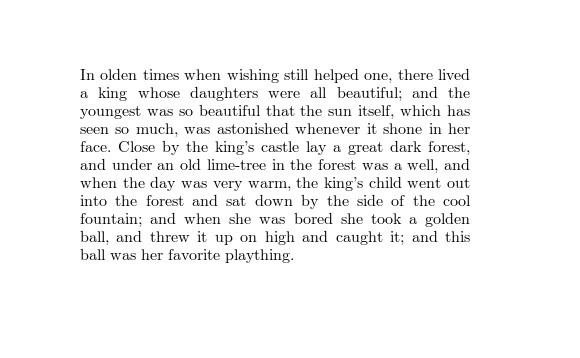There is a drawback to this approach. Because we are stretching spaces, we may end up with spaces that are so long that the text becomes difficult to read. This is particularly likely to happen if we try to make the paragraph narrower: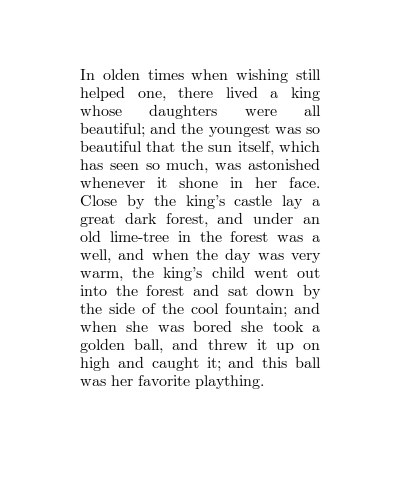The spaces between some of the words are so long, it is almost as if there are long pauses between them. If we get too many lines like that, it would actually be preferable to have shorter spaces even if the result is a ragged right edge: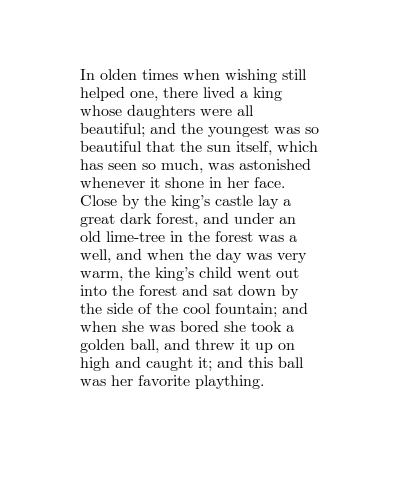This is the reason that many websites use `align: left` rather than `align: justify`. Web browsers (at least at the time of writing) don't use very sophisticated algorithms to justify text, which makes large spaces likely to occur. This is particularly true on mobile devices, which often have narrow displays.

## A More Flexible Approach

We can avoid long spaces by allowing the line breaking algorithm more freedom in where to break lines. Instead of always breaking a line before a word that doesn't fit, we may allow the algorithm to choose to break the line after a word that goes beyond the line length. This implies two things. One, we are now allowing spaces to be compressed as well as stretched. Two, we need some way to decide which alternative to pick: break before the word and stretch spaces, or break after the word and compress spaces. To make the decision, we assign a cost to stretching and compressing spaces. We then explore both options, compute the total cost (from the current line up to the end of the paragraph), and pick the cheaper option. This gives us the following result: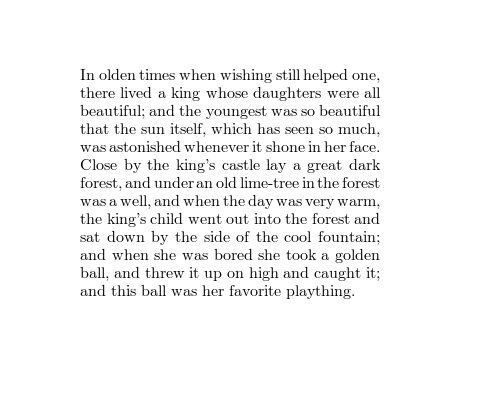Indeed, the very long spaces are gone. However, there is a new problem: some spaces have become too short, resulting in some of the words almost running together. This makes the text difficult to read. This is bad enough that we can probably just hardcode a limit on how much we are willing to compress spaces. The result is much easier to read: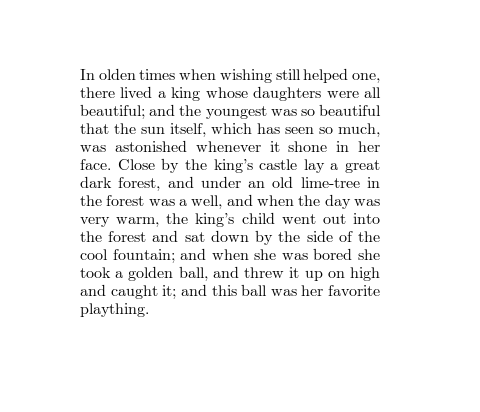### Avoiding Sudden Changes

Now that we can have long spaces as well as short spaces in a paragraph, we should try to avoid putting lines with extra long spaces next to lines with extra short spaces. The following image illustrates the problem: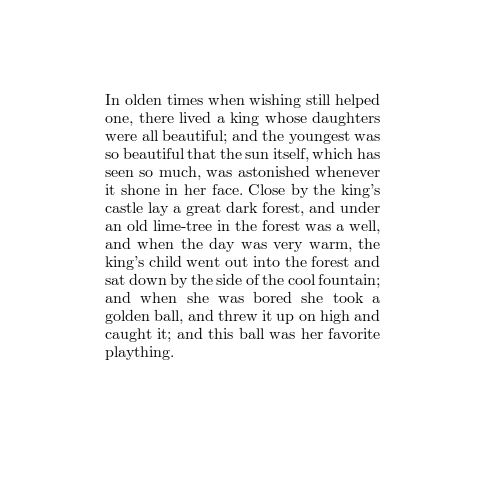The 12th line ("and when she was bored she took a") has wide spaces, whereas the lines before and after it have much narrower spaces. This makes the 12th line stand out. We can address this by adding a cost for large changes in space width. This gives us a paragraph where changes in space length are more gradual:### More Refinements

The algorithm as it stands still makes some suboptimal choices. For example, it will happily put the last word of the paragraph on a line by itself, which doesn't make for the best reading experience:We can avoid this by adding a cost to having a word on the last line by itself. This allows the algorithm to trade this off against other things. For example, depending on how we set the cost, it might decide that long spaces are better than a lonely last word:We might also add costs for other undesirable properties, such as having consecutive lines end in the same word, and a cost for consecutive lines starting with the same word.

As a finishing touch, we can adjust the tracking so that letters in words are slightly closer together or furthter apart. As long as the adjustment is small enough, it does not become bothersome, but it does allow us to use spaces that are closer to their ideal length. For example, here is the same paragraph with the same width and the same line breaks, but the second version uses tracking adjustment to keep the spaces closer to their ideal width: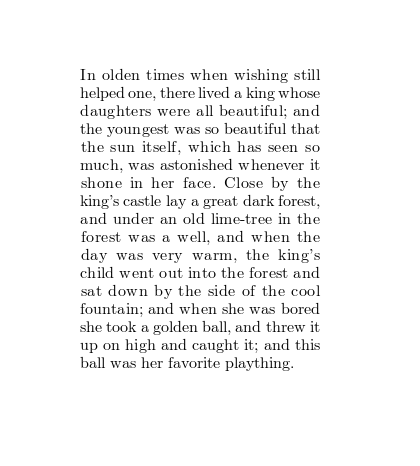The result is that long spaces are less long, short spaces are less short, and spaces have a more uniform length throughout the paragraph.

For comparison, here is the same paragraph justified to the same width, but with the first-fit algorithm from the beginning:Finally, here is a comparison to the same paragraph, typeset by LaTeX, using similar paragraph width and font size.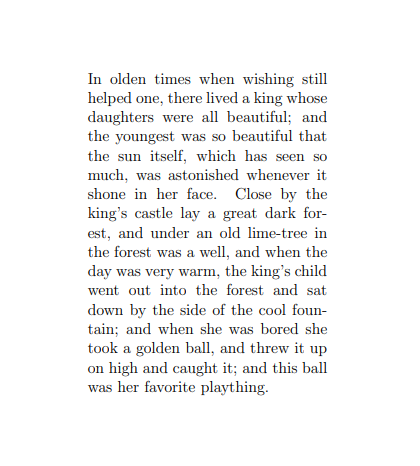## Algorithm

Here is a tarball with a small Rust project that implements the algoriths used to generate the images for this page: alinea-0.1.0.tar.bz2 (5806 bytes). `cargo run --release` can be used to run the program.

Here is the code that generates the line breaks:

break_paragraph_fancy
``````
extern create cairo;

// Cost of having only one word on the last line of the paragraph.
const solo_last_line_cost : f64 = 30.0;

// Cost of changing the length of a space between two lines.
fn space_diff_cost(old: f64, new: f64) -> f64 {
let space_change;
if old > new {
space_change = old / new;
} else {
space_change = new / old;
}
if space_change > 1.2 {
let space_change = space_change - 1.2;
10.0 * space_change * space_change
} else {
0.0
}
}

// Cost of having spaces of length space_len instead of ideal_space_len.
fn space_len_cost(space_len: f64, ideal_space_len: f64) -> f64 {
if space_len > ideal_space_len * 1.2 {
let b = (space_len - ideal_space_len * 1.2) / ideal_space_len;
20.0 * b * (b + 0.5)
} else if space_len < ideal_space_len * 0.8 {
let b = (ideal_space_len * 0.8 - space_len) / ideal_space_len;
20.0 * b * (b + 0.5)
} else {
0.0
}
}

fn break_paragraph_fancy<'a>(context: &cairo::Context,
text: &'a Vec::<&str>,
x: f64,
left_margin: f64,
right_margin: f64) -> Vec::<Vec::<&'a str>> {
// Algorithm
//
// We start by accumulating words in the first line. If we reach the
// end of the text before the line is full, we are done.
// If we find a word that does not fit in the line, we recursively
// compute the optimal solution for a text that starts with that word
// on a new line. We store that solution in the cache. Then we check
// again, this time with the word that didn't fit added to the line
// and starting the next line with the word after. We then choose the
// better of the two solutions.
// A cache entry consists of a score, a next line break position, and a
// space length for the line that starts at that position.
let mut cache = Vec::<(f64, usize, f64)>::new();
cache.resize(text.len(), (0.0, 0, 0.0));
fn abs(x: f64) -> f64 { if x < 0.0 { -x } else { x } }
fn recurse<'b>(context: &cairo::Context,
text: &'b Vec::<&str>,
i: usize,
x: f64,
left_margin: f64,
right_margin: f64,
ideal_space_len: f64,
cache: &mut Vec::<(f64, usize, f64)>) -> (f64, usize, f64) {
// Compute solution and score from starting with word i at horizontal position x.
let mut i = i;
let mut remaining = right_margin - x + ideal_space_len;
let mut spaces_in_line = 0;
loop {
let need_space = context.text_extents(text[i]).x_advance + ideal_space_len;
if need_space <= remaining {
i += 1;
spaces_in_line += 1;
remaining -= need_space;
if i >= text.len() {
// We don't want the last line to contain only a single word.
if spaces_in_line == 1 {
return (solo_last_line_cost, i, ideal_space_len);
}
// Perfect solution found!
return (0.0, i, ideal_space_len);
}
continue;
}
// We need a line break. See what happens if we break the line here...
if cache[i].1 == 0 {
let soln = recurse(context, text, i, left_margin, left_margin, right_margin, ideal_space_len, cache);
cache[i] = soln;
}
let space_adj = remaining / spaces_in_line as f64;
let space_len = ideal_space_len + space_adj;
let early = cache[i];
let early_cost = early.0 + space_len_cost(space_len, ideal_space_len) + space_diff_cost(space_len, early.2);
let early_result = (early_cost, i, space_len);
if i + 2 > cache.len() {
return early_result;
}
// Now check what happens if we break the line at the next word instead.
if cache[i + 1].1 == 0 {
let soln = recurse(context, text, i + 1, left_margin, left_margin, right_margin, ideal_space_len, cache);
cache[i + 1] = soln;
}
remaining -= need_space;
let space_adj = remaining / spaces_in_line as f64;
let space_len = ideal_space_len + space_adj;
let late = cache[i + 1];
let late_cost = late.0 + space_len_cost(space_len, ideal_space_len) + space_diff_cost(space_len, late.2);
// And pick the better solution. Avoid reducing spaces below 1/3 of their ideal width.
if late_cost < early_cost && space_adj >= -ideal_space_len * 0.3 {
return (late_cost, i + 1, space_len);
} else {
return early_result;
}
}
}
let mut soln = recurse(context, text, 0, x, left_margin, right_margin, ideal_space_len, &mut cache);
let mut lines = Vec::<Vec::<&str>>::new();
let mut i = 0;
let mut line = Vec::<&str>::new();
loop {
if i == soln.1 {
lines.push(line);
if i >= text.len() { break };
soln = cache[i];
line = Vec::<&str>::new();
}
line.push(text[i]);
i += 1;
};
return lines;
}
``````